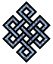#fail2ban bad ip database: ip 200.37.55.50

### | ip database | live view | stats | report | help | api key:

 ip: 200.37.55.50 hostname: 200.37.55.50 country:[PE] Peru first reported: 20.09.2018 03:47.51 GMT+0200 last reported: 25.02.2019 17:55.05 GMT+0200 time period: 158d 15h 07m 14s total reports: 12 reported by: 3 host(s) filter(s): ssh (8) ssh (4) tor exit node no badips.com db Lookup## port scan of '200.37.55.50':

[-hide]
```# Nmap 6.40 scan initiated Thu Sep 20 03:48:02 2018 as: /usr/bin/nmap -sU -sS -O 200.37.55.50
Nmap scan report for 200.37.55.50
Host is up (0.19s latency).
Not shown: 1000 open|filtered ports, 996 filtered ports
PORT    STATE  SERVICE
22/tcp  open   ssh
80/tcp  open   http
113/tcp closed ident
443/tcp closed https
No exact OS matches for host (If you know what OS is running on it, see http://nmap.org/submit/ ).
TCP/IP fingerprint:
OS:SCAN(V=6.40%E=4%D=9/20%OT=22%CT=113%CU=%PV=N%G=Y%TM=5BA2FC8B%P=x86_64-pc
OS:-linux-gnu)SEQ(SP=105%GCD=1%ISR=106%TI=Z%TS=8)OPS(O1=M5B4ST11NW7%O2=M5B4
OS:ST11NW7%O3=M5B4NNT11NW7%O4=M5B4ST11NW7%O5=M5B4ST11NW7%O6=M5B4ST11)WIN(W1
OS:=7120%W2=7120%W3=7120%W4=7120%W5=7120%W6=7120)ECN(R=Y%DF=Y%TG=40%W=7210%
OS:O=M5B4NNSNW7%CC=Y%Q=)T1(R=Y%DF=Y%TG=40%S=O%A=S+%F=AS%RD=0%Q=)T2(R=N)T3(R
OS:=N)T4(R=N)T5(R=Y%DF=N%TG=40%W=0%S=Z%A=S+%F=AR%O=%RD=0%Q=)T6(R=N)T7(R=N)U
OS:1(R=N)IE(R=N)

OS detection performed. Please report any incorrect results at http://nmap.org/submit/ .
# Nmap done at Thu Sep 20 03:48:59 2018 -- 1 IP address (1 host up) scanned in 57.91 seconds
```
```Σ = 79 | Δt = 0.0043141841888428s
```